MCAT Physical : Purification Techniques

Example Questions

← Previous 1

Example Question #1 : Distillation

Consider the following solutions.

Solution A: 1M sodium chloride solution

Solution B: 1M calcium nitrate solution

Solution C: 1M sucrose solution

Equal volumes of the solutions are combined and the mixture is added to a distillation column. Which of the following solutions will separate first?

Solution B

Solution A

Solution C

These solutions cannot be separated via distillation

Solution C

Explanation:

Distillation is a process of separating a liquid from solutes or other liquids. It utilizes the boiling point differences to separate substances. A substance with a low boiling point will evaporate first in a distillation column and will be isolated first. The question is asking which solution will be isolated first; therefore, we need to figure out which solution has the lowest boiling point. Recall that the boiling point of a solution is elevated when there are more solutes present in the solution. Sodium chloride (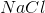) contributes two solutes (sodium ions and chloride ions). Calcium nitrate (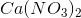) contributes three solutes (one calcium ion and two nitrate ions). Sucrose does not dissociate into ions in solution; therefore, it only contributes one solute. This means that the sucrose solution will have the lowest amount of molecules in solution, the lowest boiling point, and will be separated first.

Example Question #2 : Distillation

Small differences in boiling point require the use of __________ distillation and large differences in boiling point require the use of __________ distillation.

simple . . . simple

simple . . . fractional

fractional . . . simple

fractional . . . fractional

fractional . . . simple

Explanation:

There are two types of distillation. Simple distillation is used to separate molecules that have very different boiling points. Fractional distillation is used to separate molecules with small differences in boiling points. Fractional distillation is often used if the difference between boiling points is less than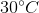. In simple distillation, the vapor is immediately collected in a condenser. On the other hand, fractional distillation allows vapor to condense and revaporize several times. These repeated cycles allow fractional distillation to purify the vapor better than simple distillation.

Example Question #3 : Distillation

Which of the following mixtures can be separated using fractional distillation (boiling points of each substance given in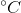)?

I. Chloroform (62.2) and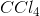(76.7)

II. Iodine (184.3) and mercury (356.9)

III. Nitric acid (120) and sulfuric acid (310)

I only

I, II, and III

II only

II and III

I, II, and III

Explanation:

Distillation is used to separate molecules with different boiling points. Simple distillation is used to separate molecules with vastly different boiling points. Fractional distillation, on the other hand, is a refined form of simple distillation that can be used to separate molecules with similar boiling points. Note that fractional distillation can separate molecules with either different or similar boiling points; therefore, fractional distillation can be used to separate any of the given mixtures.

Example Question #4 : Distillation

Which of the following conditions will result in the greatest increase in the rate of distillation of a substance?

Decreasing the mole fraction of the substance

Decreasing the vapor pressure

Decreasing the atmospheric pressure

Decreasing the temperature

Decreasing the atmospheric pressure

Explanation:

Rate of distillation is increased when the ability of a substance to become a vapor is increased. Recall that vapor is created when enough heat is applied to the liquid. The temperature at which the liquid becomes vapor is called the boiling point. A liquid turns into a vapor when the vapor pressure (pressure applied by the vapor from the liquid) equals the atmospheric pressure. Decreasing the atmospheric pressure will make it easier for the liquid to turn into a vapor; therefore, this will increase the rate of distillation.

Decreasing the vapor pressure will remove vapor from system. This will make it harder to distill substances. Decreasing temperature will move the system away from the boiling point, thereby decreasing the amount of vapor. Decreasing mole fraction of the substance will decrease the surface area of the substance (at the surface of the solution). Liquid molecules need to be present at the surface to escape the solution and become vapor; therefore, decreasing mole fraction will decrease the amount of vapor.

Example Question #1 : Thin Layer Chromatography

A new student is planning to use thin layer chromatography (TLC) for his research project. After setting up the apparatus the student forgets to place a lid on the TLC jar. He obtains poor results after running the TLC experiment. Which of the following can best explain his bad results?

The open system prevents the evaporation of the solvent on the TLC plate

The open system evaporates the solvent on the TLC plate

The open system evaporates the solvent in the solution

The open system prevents the evaporation of the solvent in the solution

The open system evaporates the solvent on the TLC plate

Explanation:

TLC is a laboratory technique commonly used to separate components of a mixture. Mixtures are placed on the TLC plate (stationary phase), which is then transferred to a jar containing the solvent. The solvent travels through the plate and carries components of the mixture along with it. Based on its properties, each component is dragged to different distances on the plate. The relative distances travelled by each component can be used for separation and identification.

It is important to place a lid on the jar because the solvent will be a volatile substance. An open system will allow for the solvent to evaporate from the TLC plate and reduce the amount of solvent travelling through the plate. The solvent in the solution will evaporate, but it is negligible and inconsequential to the data collected on the TLC plate.

Example Question #2 : Thin Layer Chromatography

Which of the following can be determined from thin layer chromatography results?

I. Number of components in the mixture

II. The identity of the components

III. Polarity of components

I, II, and III

II only

I and III

II and III

I and III

Explanation:

Thin layer chromatography (TLC) is used to separate components in a mixture. Components are separated on a TLC plate because each component travels a different distance. The distance travelled depends on several factors. One of those factors is polarity; therefore, TLC can used to determine polarity of substances.

TLC is not useful for identifying substances. Other techniques such as NMR and IR spectroscopy are useful for identifying individual components. The Rf value of a compound can help identify it in a broad sense, but this technique provides only very rudimentary identification capabilities.

Example Question #3 : Thin Layer Chromatography

After performing a TLC experiment, a researcher determines the Rf value of a component to be 2. He also notes that the solvent travelled a distance of 4cm on the plate. What can you conclude about this experiment?

There are multiple components in the mixture

The reported data does not seem valid

The component travelled a distance of 8cm

The solvent is not volatile

The reported data does not seem valid

Explanation:

To solve this problem we need to recall the definition of Rf.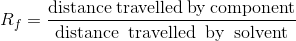The solvent is typically the most mobile substance on a TLC plate; therefore, it travels the farthest distance. This means that Rf is always less than one because the numerator of Rf is always smaller than the denominator. The question states that the Rf value is 2. Since this is greater than 1, the results don’t seem valid. This value indicates that the solute compounds were able to teravel farther on the TLC plate than the solvent (mobile phase).

Example Question #4 : Thin Layer Chromatography

Compounds A and B are found in a mixture. Rf values for both compounds were calculated. Which of the following sets of Rf values would reflect the largest separation between A and B?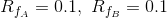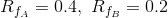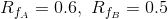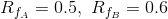Explanation:

Compounds A and B are in the same mixture; therefore, they will be separated using the same TLC plate. Recall the definition of Rf.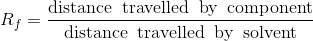Since the same TLC plate was used, the denominator for both compound A and B will be the same (the total distance travelled by solvent alone is the same for a TLC plate). To separate them effectively, compounds must have travelled different distances along the plate. The best separation occurs when the difference between the distances travelled by the compounds is greatest.

Let’s assume the denominator to be 1 for both compounds. This means that the numerator is equal to the Rf value. If we compare the given Rf values with each other we realize that Rf values of 0.4 and 0.2 will give the biggest difference between the distances travelled by compounds A and B (difference is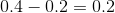); therefore, this will have the best separation.

Example Question #1 : Electrophoresis

In gel electrophoresis, a negatively charged particle will migrate towards the __________ and a positively charged particle will migrate towards the __________

anode . . . anode

cathode . . . anode

cathode . . . cathode

anode . . . cathode

anode . . . cathode

Explanation:

Gel electrophoresis is a technique used to separate molecules based on size or charge. Charged particles can be separated because they migrate towards different ends of the gel.

Negatively charged particles always migrate towards the positive pole whereas positively charged particles always migrate towards the negative pole (opposites attract). In gel electrophoresis, the positive pole is called the anode and the negative pole is called the cathode; therefore, the charged particles will migrate to the respective nodes.

Example Question #2 : Electrophoresis

Which of the following is true regarding the isoelectric point (pI)?

I. It is a property of both the species and the environment

II. The environment is basic if the pH is greater than the pI

III. It represents the size of the species

III only

I and II

II and III

II only